Friday, 30 November 2001 00:00

## Using Concatenate Function in Excel

Written by
Rate this item
(1 Vote)
using-concatenate-function-in-excel

## Excel CONCATENATE function

The CONCATENATE function in Excel allows different pieces of text to join into one or combine values from several cells into one cell.

The syntax of Excel CONCATENATE i:

CONCATENATE(text1, [text2], …)

Where text is a text string, cell reference or formula-driven value.

The following examples show the CONCATENATE FUNCTION in action:

#### Example 1: Concatenating the values of several cells: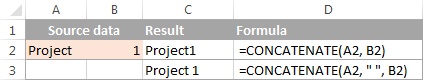In the example above the EXCEL CONCATENATE function joins the value of CELL A1 (the string project") with the Value of CELL B (The string 1) into the CELL C1. Cell D1 shows the formula used.

#### Example 2: Concatenating a text string and cell value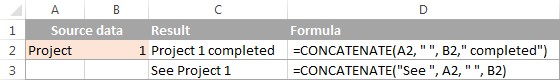The above example demonstrates that Excel CONCATENATE function is not limited to cell values; it can also concatenate various text strings.

`=CONCATENATE(A1, " ", B1, " completed")`

The above first formula of which the results is diplayed in cell D1 shows that the value of Cell A1 is merged with the value of Cell B1 and the word or string "completed".

`=CONCATENATE("See ", A1, " ", B1)`

In the second formula a space (" ") is added in between the combined values, so that the result displays as "Project 1" rather than "Project1".

#### Concatenating a text string and a formula-calculated value

To make the result returned by some formula more understandable for your users, you can concatenate it with a text string that explains what the value actually is.

For example, you can use the following formula to return the current date:

`=CONCATENATE("Today is ",TEXT(TODAY(), "dd-mmm-yy"))`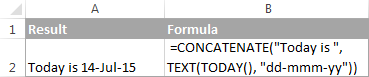## "&" operator to concatenate strings in Excel

Another way to concatenate cells in Microsoft Excel, is to use  & operator. This method can be helpful as it is a substantial gain of time because typing the ampersand sign (&) is quicker than typing the word "concatenate" ?

The & operator works in the same way as the CONCATENATE function; it combines different text strings, cell values and results returned by other functions.

#### Example 3: using Excel "&" formula examples

The following formulas show how to use the & operator:

Concatenate the values in A1 and B1:
`=A1&B1`

Concatenate the values in A1 and B1 separated with a space:
`=A1&" "&B1`

Concatenate the values in A1, B1 and a text string:
`=A1 & B1 & " completed"`

Concatenate a string and the result of the TEXT / TODAY function:
`="Today is " & TEXT(TODAY(), "dd-mmm-yy")`

Below the pictures shows that the CONCATENATE function and "&" operator return identical results: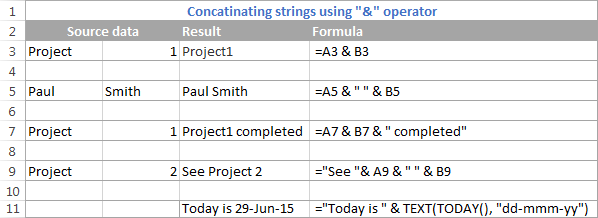## Concatenate cells with a space, comma and other characters

In your worksheets, you may often need to join valuesto include commas, spaces, punctuation marks or other characters such as a hyphen or slash. To do this, simply include the character you want in your concatenation formula and enclose that character in speech marks, as demonstrated in the following examples.

Concatenating two cells with a space:

`=CONCATENATE(A1, " ", B1)` or `=A1 & " " & B1`

Concatenating two cells with a comma:

`=CONCATENATE(A1, ", ", B1)` or `=A1 & ", " & B1`

Concatenating two cells with a hyphen:

`=CONCATENATE(A1, "-", B1)` or `=A1 & "-" & B1`

The following screenshot demonstrates how the results may look like: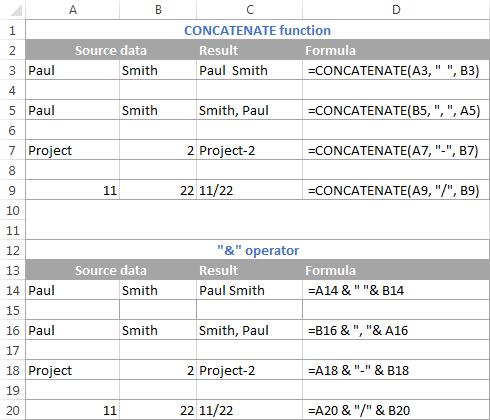## Concatenate text strings with line breaks

Most often, you would separate the concatenated text strings with punctuation marks and spaces, as shown in the previous example. In some cases, however, may need to separate the values with a line break, or carriage return. A common example is merging mailing addresses from data in separate columns.

A problem is that you cannot simply type a line break in the formula like a usual character, and therefore a special CHAR function is needed to supply the corresponding ASCII code to the concatenation formula:

• On Windows, use CHAR(10) where 10 is the ASCII code for Line feed.
• On the Mac system, use CHAR(13) where 13 is the ASCII code for Carriage return.

In this example, we have the address pieces in columns A through F, and we are putting them together in column G by using the concatenation operator "&". The merged values are separated with a comma (", "), space (" ") and a line break CHAR(10):

`=A2 & " " & B2 & CHAR(10) & C2 & CHAR(10) & D2 & ", " & E2 & " " & F2`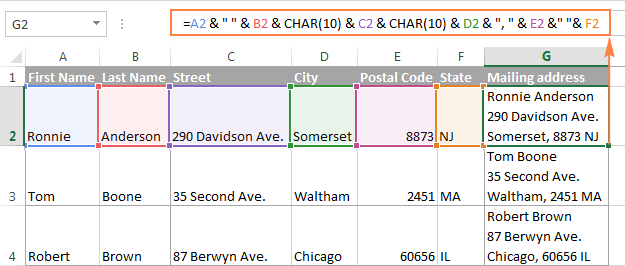Note. When using line breaks to separate the concatenated values, you must have the "Wrap text" option enabled for the result to display correctly. To do this, press Ctrl + 1 to open the Format Cells dialog, switch to the Alignment tab and check the Wrap text box.

In the same manner, you can separate concatenated strings with other characters such as:

• Double quotes (") - CHAR(34)
• Forward slash (/) - CHAR(47)
• Asterisk (*) - CHAR (42)
• The full list of ASCII codes is available

Though, an easier way to include printable characters in the concatenation formula is to simply type them in double quotes as we did in the previous example.

Either way, all four of the below formulas yield identical results:

`=A1 & CHAR(47) & B1`

`=A1 & "/" & B1`

`=CONCATENATE(A1, CHAR(47), B1)`

`=CONCATENATE(A1, "/", B1)`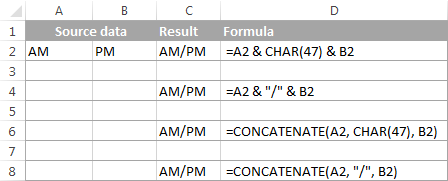## How to concatenate columns in Excel

In order to concatenate two or more columns in Excel, you just enter a usual concatenation formula in the first cell, and then copy it down to other cells by dragging the fill handle (the small square that appears in the lower right hand corner of the selected cell).

For example, to concatenate two columns (column A and B) separating the values with a space, you enter the following formula in cell C2, and then copy it down to other cells. When you are dragging the fill handle to copy the formula, the mouse pointer changes to a cross, as shown in the screenshot below: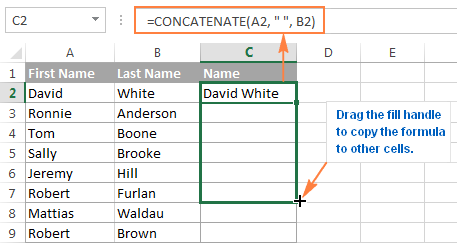Tip. A quick way to copy the formula down to other cells in the column is to select the cell with the formula and double-click the fill handle.

Please note that Microsoft Excel determines how far to copy cells after the fill handle double click based on the cells referred to by your formula. If there happen to be empty cells in your table, say cell A6 and B6 were blank in this example, the formula would be copied up to row 5 only. In this case, you would need to drag the fill handle down manually to concatenate the entire columns.

Save

Save